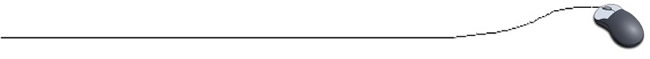Sample Fragments using the for loop

 // Counting from 10 to 50 in increments (steps) of 5: int x; for ( x = 10; x <= 50; x = x + 5 )    //**increment/decrement won't work here. {                                                 // x = x + 5 is same as x += 5      System.out.println("Loop counter value is " +  x ); }//Counting from 50 to10 backwards in increments of 5: int x; for ( x = 50; x >= 10; x = x - 5 )   // x = x - 5 is same as x -=5 {      System.out.println("Loop counter value is " + x); }// Declaring and assigning the startExpression within the for loop: //Counting down from 10 to Blast Off for ( int i = 10; i >= 1; i - - )     // notice the int {      System.out.println( i ); } System.out.println("Blast off!");// Keeping track of a "total" of values using a for loop: int total = 0;    // must be initialized before the loop (avoid "garbage") for (int count = 5; count <= 10; count ++ ) {      total += count; } System.out.println("The total is " + total);// The body of the loop may contain statements that further check on data:  for (int ctr = 1; ctr <= 35; ctr++)    // will address 35 children {      System.out.println("Enter the child's name: ");      child = reply.nextLine();      System.out.println("Enter the child's age: ");      age = reply.nextInt();      if ((age >= 6) && (age <= 7))      {           System.out.println(child + " has Mr. Escalante for a teacher.");      }      else      {            System.out.println(child + " does not have Mr. Escalante for a teacher.");      } }    // Quits after 35 times.// User determines length of for loop: System.out.println("How many stars shall i print?"); int num = reply.nextInt(); System.out.println("OK! Here I go .... "); for (i = 1; i <= num; i++) {      System.out.print( * + " "); }// Titles and for loops: //print titles OUTSIDE the loop to prevent multiple copies of the title //print even numbers from 1 to 20 with title System.out.println("Even numbers from 1 to 20 ");  //title is outside loop for (int num = 2; num <= 20; num += 2)   //notice startExpression {      System.out.print( num + " ") ;    //prints the even numbers }// Using a Flag //a "flag" is used to indicate that something happened within the program //that will effect the rest of the program in some manner int flag = 1; System.out.println("Enter a name to be examined. "); String name = reply.nextLine(); for (i = 0; i < name.length(); i++)   //i represents indices of the String {      if (name.charAt(i) == 'e')           flag = 0; //flag is set if the letter "e" is encountered } // Use the flag if (flag == 0)      System.out.println("found an \"e\"");